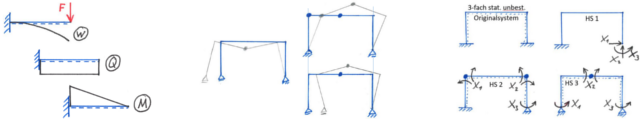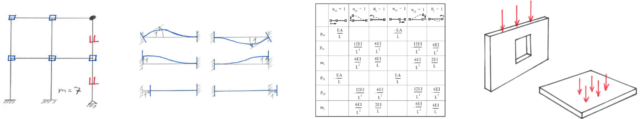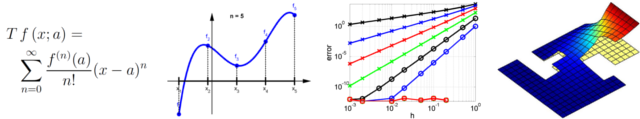# Structural Analysis 1

The aim of structural analysis is to predict the behavior of structures. In particular, deformations and stresses due to a certain loading are derived. The course Structural Analysis 1 is dedicated to structures which consists of beams and trusses. Statically determinate and indeterminate systems are calculated by conventional methods of analysis by hand. For statically indeterminate systems, the flexibility method (force method) is taught. The principles of virtual work and virtual forces are intensively discussed.# Structural Analysis 2

Displacement methods are introduced in Structural Analysis 2. They enable an efficient calculation of displacements and stresses in beam structures. Moreover, these methods serve as preliminary for computational structure analysis. Corresponding computer implementations are detailed (in Matlab®). Selected topics in structural analysis are covered: influence lines, three-dimensional systems, load factor or limit state analysis. Plates and shells are introduced as important elements in structural engineering. The theoretical models and their properties are discussed and their approximate solution based on the finite element method (FEM) is outlined.# Mathematics 3

This course consists of two parts: "Statistics and probability theory" on the one hand and "Numerics of differential equations" on the other, the latter is covered by the Institute of Structural Analysis. The solution of differential equations is an essential part in many applications in civil engineering. In most cases, exact solutions can not be provided so that the solution must be approximated. Therefore, numerical methods (simulation methods) are used, typically leading to a large system of equations for the unknown solution at selected points in the domain of interest. This course gives attention to the finite difference method (FDM) where differential operators are replaced by finite differences. Typical models in civil engineering such as beams, rods, and shells are solved by the FDM. In particular, static/dynamic and linear/non-linear situations are outlined. For the implementations, Matlab® is used which is introduced within this course.